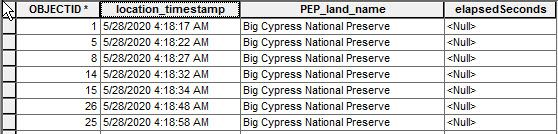# updateCursor row[n+1] from searchCursor row[n]-row[n+1]

436
10
02-08-2021 12:10 PMbyMVP Frequent Contributor

FileGDB
ArcGIS 10.4

Need to calculate elapsedSeconds column by first sorting on location_timestamp and then evaluate if PEP_land_name matches in row[n] and row[n+1]. If true, then set row[n+1] to elapsedSeconds value.

``````fields = ['PEP_land_name','location_timestamp','elapsedSeconds']
with arcpy.da.UpdateCursor(tracksToProcMonth, fields, sql, sql_clause=(None, 'ORDER BY location_timesatmp ASC')) as u_cur:
with arcpy.da.SearchCursor(tracksToProcMonth,fields, sql, sql_clause=(None, 'ORDER BY location_timestamp ASC')) as cursor:
for row in cursor:
firstlLand = row
firstTimestamp = row
try:
nextLand = cursor.next()
nextTimestamp = cursor.next()

if firstlLand == nextLand:
tdt = nextTimestamp - firstTimestamp
totSecs = tdt.total_seconds()
print 'totSecs: {}'.format(totSecs)
u_row = totSecs
u_cur.updateRow()

else:
totSecs = 0.00
u_row = totSecs
u_cur.updateRow()``````I seem to be close in that I can arrive at the correct sort and evaluation, but I'm not exactly sure what to do about the updateCursor and setting the elapsedSeconds value in row[n+1].  In the attached image, OID 1 elapsedSeconds would be 0, OID 5 would be 5, and so on and on...

1 Solution

Accepted SolutionsbyMVP Frequent Contributor

Unless I've misinterpreted what you're trying to achieve, I think it can be done without and .next() or nested cursors.

I've just created global variables to hold the previous land and timestamp values for each row:

``````import arcpy

fc = r'PathtoMyFC'
previous_timestamp = ''
previous_land = ''

with arcpy.da.UpdateCursor(fc, ['landname', 'timestamp', 'elapsed']) as cursor:
for row in cursor:

this_timestamp = row
current_land = row

if previous_timestamp is not None and current_land == previous_land:
time_diff = this_timestamp - previous_timestamp
total_seconds = time_diff.total_seconds()
row = total_seconds

previous_timestamp = this_timestamp
previous_land = current_land
cursor.updateRow(row)``````10 RepliesbyMVP Regular Contributor

u_cur.updateRow(row)  Might be what you are after?

... You can move it out from the if/else conditionals because you are setting u_row either way.

``````        if firstlLand == nextLand:
tdt = nextTimestamp - firstTimestamp
totSecs = tdt.total_seconds()
print 'totSecs: {}'.format(totSecs)
u_row = totSecs

else:
totSecs = 0.00
u_row = totSecs

u_cur.updateRow(u_row)``````byMVP Frequent Contributor

if the rows oid=1 and oid=5 (see image in op) are the 2 rows being evaluated and elapsedSeconds calculated, does your suggestion update row OID=5?

In the image OID=1 should be 0.00 seconds and OID=5 should be 5.00 seconds

Thanks!byMVP Regular Contributor

The first thing I saw from your code sample was u_row missing from the .updateRow().  I can't really answer your question without running it through the debugger and verifying that the rest of your code is valid. I can say that it will update whichever row 'u_row' is currently on.byMVP Frequent Contributor

right... I think that's my problem: I cannot update row[n+1] rather than the current row.byMVP Regular Contributor

You could probably work the values into a dictionary and do the processing outside of the cursors and then come back to update the values by OID.  I can work up a sample latter today if that is feasible.byMVP Frequent Contributor

I'm also incorrectly implementing cursor.next()

It seems each time that is invoked it immediately skips to the next row.  I'm not exactly sure how best to get the values for row n and n+1 WHILE the current row is at n.byMVP Frequent Contributor

Unless I've misinterpreted what you're trying to achieve, I think it can be done without and .next() or nested cursors.

I've just created global variables to hold the previous land and timestamp values for each row:

``````import arcpy

fc = r'PathtoMyFC'
previous_timestamp = ''
previous_land = ''

with arcpy.da.UpdateCursor(fc, ['landname', 'timestamp', 'elapsed']) as cursor:
for row in cursor:

this_timestamp = row
current_land = row

if previous_timestamp is not None and current_land == previous_land:
time_diff = this_timestamp - previous_timestamp
total_seconds = time_diff.total_seconds()
row = total_seconds

previous_timestamp = this_timestamp
previous_land = current_land
cursor.updateRow(row)``````byMVP Frequent Contributor

very promising.

...and simple.  Love that.

Let me give it a go and check back but def looks preferable to nested cursors.

Thanks,

jbyMVP Frequent Contributor

I will go ahead and mark as the solution because it appears to work as intended and I am running into another issue that needs resolved in order to actually implement this.

Thanks again!